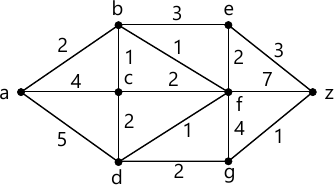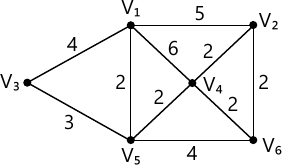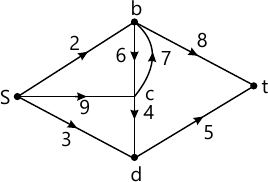MORE IN Discrete Mathematics
SPPU Computer Engineering (Semester 3)
Discrete Mathematics
December 2013
Total marks: --
Total time: --
INSTRUCTIONS
(1) Assume appropriate data and state your reasons
(2) Marks are given to the right of every question
(3) Draw neat diagrams wherever necessary

Answer any one question from Q1 and Q2
1 (a) With the help of mathematical induction prove that 8n 3n is multiple of 5, for n ≥ 1.
4 M
1 (b) What is multiset? Explain its significance with at least two examples.
2 M
1 (c) Find the transitive closure by using Warshall's algorithm A = {1,2,3,4,5,6} and R = {(x,y) | |xy| = 2}.
6 M

2 (a) Salad is made with combination of one or more eatables, how many different salads can be prepared from onion, carrot, cabbage, and cucumber?
2 M
2 (b) It is known that in university 60% of professors play tennis, 50% of them play bridge, 70% jog, 20% play tennis and bridge, 40% play bridge and jog and 30% play tennis and jog. If someone claimed that 20% professors jog and play tennis and bridge, would you believe his claim? Why?
4 M
2 (c) Show that the set of all divisors of 70 forms a lattice.
6 M

Answer any one question from Q3 and Q4
3 (a) Define the following terms with suitable example:
Group.
Monoid.
Isomorphism.
6 M
3 (b) List and explain the necessary & sufficient conditions for Hamiltonian and Eulerian path with suitable examples.
6 M

4 (a) Define the following terms with suitable example:
Ring.
Integral domain.
Field
6 M
4 (b) Find the shortest path between a-z for the graph given in figure 1 using Dijkstra's algorithm.6 M

Answer any one question from Q5 and Q6
5 (a) Define the following terms with reference to tree:
Rooted tree.
Optimal Binary tree.
Height of the tree
6 M
5 (b) Find minimum spanning tree for graph given in figure 2 using Kruskal's algorithm.7 M

6 (a) Define the following terms with reference to the tree
Binary Search Tree.
M-ary Tree.
Tree Traversal.
6 M
6 (b) Find the Maximum flow of the given Transport network in figure 3.7 M

Answer any one question from Q7 and Q8
7 (a) In a college 25% students failed in Maths, 15% student failed in Physics and 10% students failed in both Maths and Physics. A student is selected randomly then what is the probability that
i) If he failed in Physics, he also failed in Maths.
ii) He failed in maths or Physics.
6 M
7 (b) A problem on probability is given to four students A,B,C,D. The probability of solving that problem are 1/2, 3/4, 1/4 and 2/5 respectively. What is the probability that
i) The problem will be solved.
ii) Exactly one of them will solve the problem.
7 M

8 (a) Find the number of arrangements that can be made out of the letters:
i) ASSASSINATION.
ii) MISSISSIPPI
6 M
8 (b) Two cards are drawn at random from an ordinary deck of 52 cards. Find the probability that